Question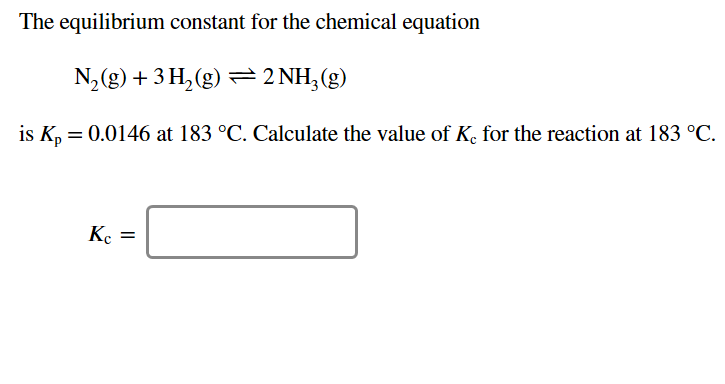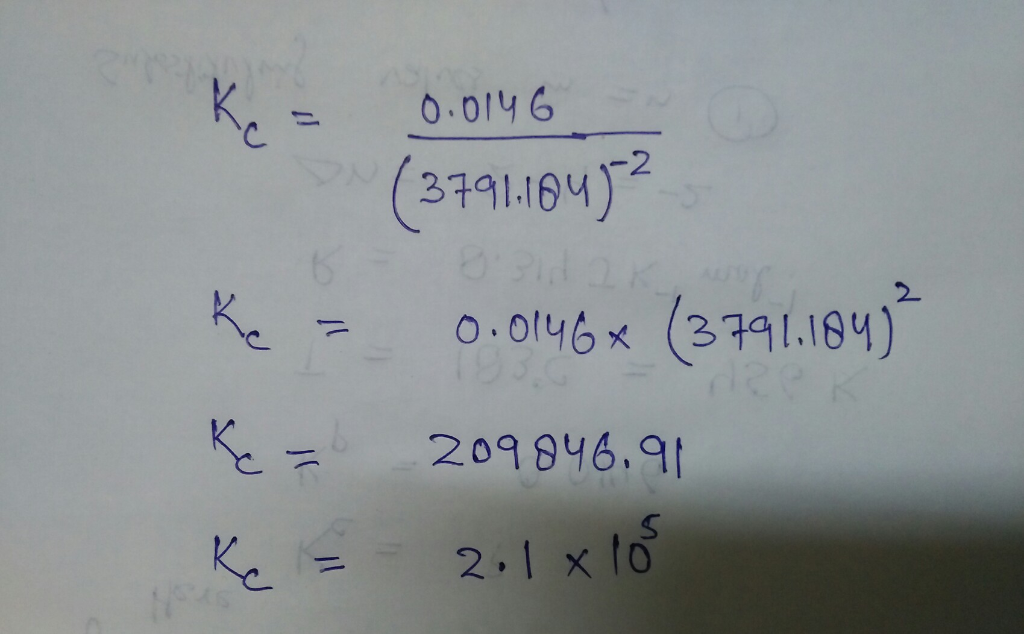#### Earn Coins

Coins can be redeemed for fabulous gifts.

Similar Homework Help Questions
• ### The equilibrium constant for the chemical equation N2(g)+3H2(g)−⇀↽−2NH3(g) is Kp=0.111 at 231 ∘C. Calculate the value...

The equilibrium constant for the chemical equation N2(g)+3H2(g)−⇀↽−2NH3(g) is Kp=0.111 at 231 ∘C. Calculate the value of Kc for the reaction at 231 ∘C.

• ### The equilibrium constant for the chemical equation N2 g) +3H 2(g) 근 2NH3(g) is Kp =...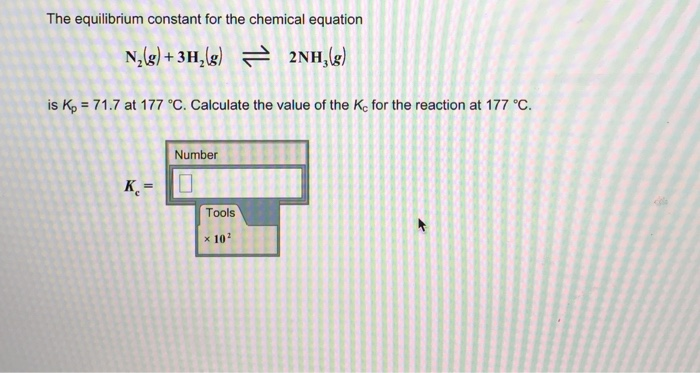The equilibrium constant for the chemical equation N2 g) +3H 2(g) 근 2NH3(g) is Kp = 71.7 at 177 °C. Calculate the value of the Ke for the reaction at 177 °C. Number K,= Tools x 102

• ### The equilibrium constant for the chemical equation N,(g) + 3H2(8) - 2NH3(g) is Kp = 0.0881...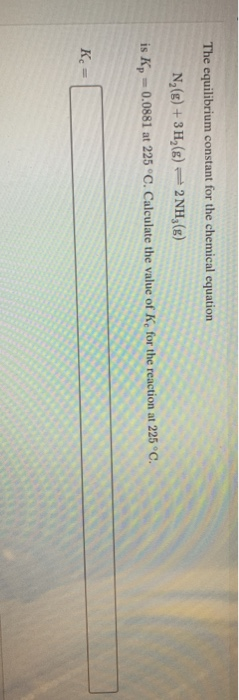The equilibrium constant for the chemical equation N,(g) + 3H2(8) - 2NH3(g) is Kp = 0.0881 at 225 °C. Calculate the value of K, for the reaction at 225°C. Kc =

• ### 2. At 55°C, the equilibrium constant for the reaction N2(g) + 3H2(g) + 2NH3(g) is Kp=0.05....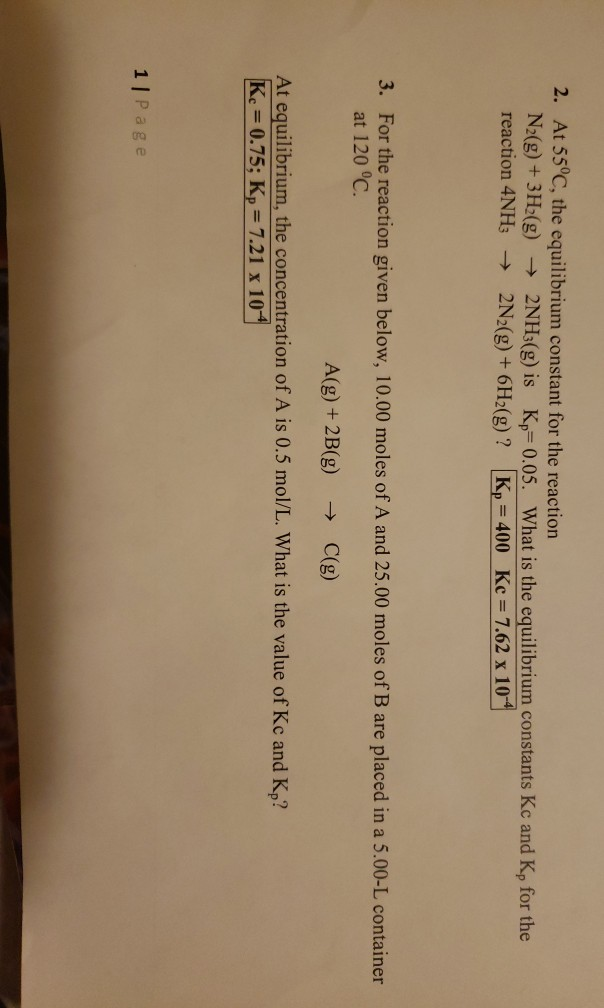2. At 55°C, the equilibrium constant for the reaction N2(g) + 3H2(g) + 2NH3(g) is Kp=0.05. What is the equilibrium constants Kc and Kp for the reaction 4NH3 + 2N2(g) + 6H2(g)? Kp = 400 Kc = 7.62 x 10 3. For the reaction given below, 10.00 moles of A and 25.00 moles of B are placed in a 5.00-L container at 120 °C. A(g) +2B(g) → C(g) At equilibrium, the concentration of A is 0.5 mol/L. What is the...

• ### Write equilibrium constant expressions, Kp, for the following reactions. i) N2(g) + 3 H2(g) ⇌ 2NH3(g)...

Write equilibrium constant expressions, Kp, for the following reactions. i) N2(g) + 3 H2(g) ⇌ 2NH3(g) ii) NH4HS(s) ⇌ NH3(g) + H2S(g)

• ### The equilibrium constant for the chemical equation N2(g)+3H2(g)↽−−⇀2NH3(g) is ?p=0.0752 at 221 ∘C. Calculate the value...

The equilibrium constant for the chemical equation N2(g)+3H2(g)↽−−⇀2NH3(g) is ?p=0.0752 at 221 ∘C. Calculate the value of ?c for the reaction at 221 ∘C.

• ### Consider the chemical reaction 2NH3(g) ó N2(g) + 3H2(g). The equilibrium is to be established in...

Consider the chemical reaction 2NH3(g) ó N2(g) + 3H2(g). The equilibrium is to be established in a 50.0 L container at 1,000 K, where Kc = 4.0 × 10-2. Initially, 6.10 x 105 moles of NH3(g) are present. Calculate the amount of H2 presentat equilibrium. [H2] =_____

• ### The equilibrium constant, Kc, for the following reaction is 6.30 at 723K. 2NH3(g) N2(g) + 3H2(g)...

The equilibrium constant, Kc, for the following reaction is 6.30 at 723K. 2NH3(g) N2(g) + 3H2(g) If an equilibrium mixture of the three gases in a 15.7 L container at 723K contains 0.284 mol of NH3(g) and 0.437 mol of N2, the equilibrium concentration of H2 is__________ M.

• ### For the reaction: 2NH3(g) = N2(g) + 3 H2(g), Kc = 3.82 x 10-8 at a...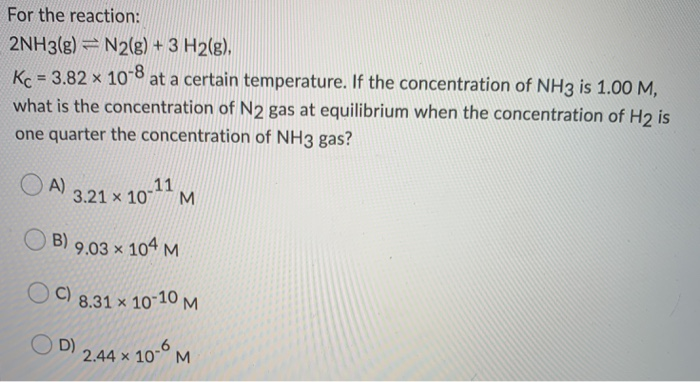For the reaction: 2NH3(g) = N2(g) + 3 H2(g), Kc = 3.82 x 10-8 at a certain temperature. If the concentration of NH3 is 1.00 M, what is the concentration of N2 gas at equilibrium when the concentration of H2 is one quarter the concentration of NH3 gas? OA) 3.21 x 10-11 M OB) 9,03 104 M OC) 8.31 * 10-10 M OD) 2.44 x 10-6M

• ### I've tried this a million times and I keep getting it wrong! The equilibrium constant for...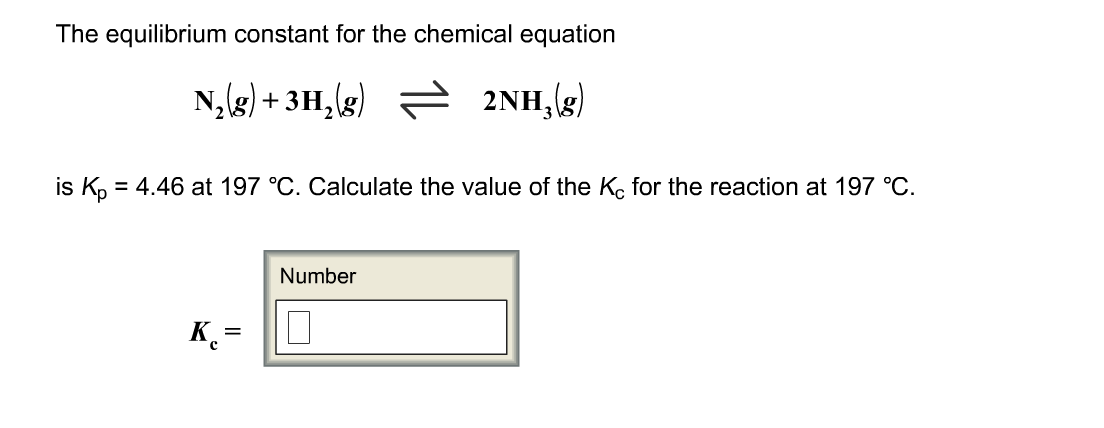I've tried this a million times and I keep getting it wrong! The equilibrium constant for the chemical equation N2(g)+3H2(g)2NH3(g) s Kp = 4.46 at 197 degree C. Calculate the value of the K for the reaction at 197 degree C. Kc =